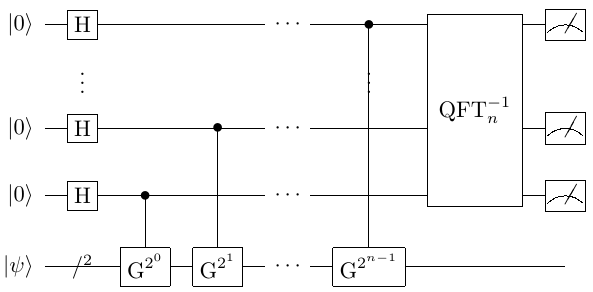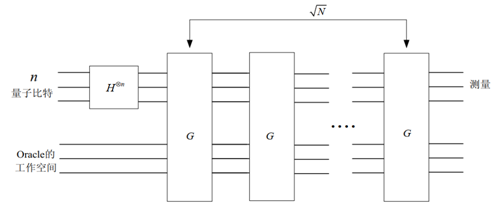# Grover算法和量子计数算法¶

## 问题背景概述¶

\begin{aligned} \left|\psi\right\rangle=sin\theta\left|\varphi_1\right\rangle+cos\theta\left|\varphi_0\right\rangle, \ \left|\varphi_0\right\rangle=\left|\varphi_1^\bot\right\rangle. \end{aligned}

### 量子计数问题¶

$$\left|\Omega\right|=N=2^n,\Omega\supseteq B, \left|B\right|=M\le N$$，且判别函数满足

\begin{split}\begin{aligned} \left\{\begin{matrix} f:\Omega\rightarrow\{0,1\},\\ f\left(x\right)=\left\{\begin{matrix} 1,x\in B,\\ 0,x\notin B. \end{matrix}\right. \end{matrix}\right. \end{aligned}\end{split}

$$M$$

### 解元素的搜索问题¶

\begin{split}\begin{aligned} \left\{\begin{matrix} f:\Omega\rightarrow\{0,1\},\\ f\left(x\right)=\left\{\begin{matrix} 1,x=\omega,\\ 0,x \neq \omega. \end{matrix}\right. \end{matrix}\right. \end{aligned}\end{split}

$$\omega \in \Omega$$

Grover算法的过程与振幅放大量子线路的过程完全一致。Grover算法的时间复杂度为 $$O(\sqrt N)$$，相对于经典算法的O(N)有着极大加速。

## 算法原理¶

\begin{aligned} \left|\psi\right\rangle=sin\theta\left|\varphi_1\right\rangle+cos\theta\left|\varphi_0\right\rangle, \ \left|\varphi_0\right\rangle=\left|\varphi_1^\bot\right\rangle. \end{aligned}

### 基于振幅放大算子的QPE过程¶

\begin{aligned} \left|\varphi_0\right\rangle=\frac{1}{\sqrt{N-M}}\sum_{x\notin B}\left|x\right\rangle, \left|\varphi_1\right\rangle=\frac{1}{\sqrt M}\sum_{x\in B}\left|x\right\rangle. \end{aligned}

\begin{aligned} G(\cos{\theta}\left|\varphi_0\right\rangle+\sin{\theta}\left|\varphi_1\right\rangle) =\cos{3\theta}\left|\varphi_0\right\rangle+\sin{3\theta}\left|\varphi_1\right\rangle. \end{aligned}

$$G$$ 的特征值为 $$e^{\pm2i\theta}$$，借助在制备 $$\psi$$ 的过程中使用的索引比特，可以准确区分出以 $$G$$ 构造的QPE过程结果对应的特征子相位是 $$2\theta$$$$2\pi-2\theta$$

### 基于镜像变换的振幅放大量子线路¶

\begin{split}\begin{aligned} U_\omega=(I-2\left|\omega\right\rangle\langle\omega|)=\left[\begin{matrix}-1&-\frac{2}{\sqrt N}\\0&1\\\end{matrix}\right], U_s=2\left|\varphi\right\rangle\langle\varphi|-I=\left[\begin{matrix}-1&0\\\frac{2}{\sqrt N}&1\\\end{matrix}\right]. \end{aligned}\end{split}

$$\sin{\theta}=\frac{1}{\sqrt N},a=e^{i\theta},\ \ \frac{1}{\sqrt N}=\frac{a-a^{-1}}{2i}$$，于是有

\begin{split}\begin{aligned} U_\omega U_s=\frac{1}{a^2+1}\left[\begin{matrix}-i&i\\a&a^{-1}\\\end{matrix}\right]\left[\begin{matrix}a^2&0 \\0&a^{-2}\\\end{matrix}\right]\left[\begin{matrix}i&a\\-a^2i&a\\\end{matrix}\right]. \end{aligned}\end{split}

$$Q=U_sU_\omega$$ ，有 $$Q\left|\varphi\right\rangle=\frac{N-4}{N}\left|\varphi\right\rangle +\frac{2}{\sqrt N}\left|\omega\right\rangle$$ ，且

$\begin{split}Q^k = \frac{1}{a^2+1}\left[\begin{matrix}-i&i\\a&a^{-1}\\\end{matrix} \right]\left[\begin{matrix}a^{2k}&0\\0&a^{-2k}\\\end{matrix}\right]\left[\begin{matrix}i&a\\-a^2i&a\\\end{matrix}\right].\end{split}$

$$\left|\varphi\right\rangle$$ 执行量子门 $$Q^k$$ 后，测量第一个寄存器得到解量子态 $$\left|\omega\right\rangle$$ 的概率为 $$P(\omega)={\langle\omega|Q}^k\left|\varphi\right\rangle=\left[\begin{matrix}\left\langle\omega|\omega\right\rangle &\left\langle\omega|\varphi\right\rangle\\\end{matrix}\right]{{{(U}_sU}_\omega)}^k\left[\begin{matrix}0\\1\\\end{matrix}\right] =\frac{a^{2k+1}-a^{-\left(2k+1\right)}}{2i}=\sin{(\left(2k+1\right)\theta)}$$

$$\left(2k+1\right)\theta=\frac{\pi}{2}$$ 可知经过 $$k=[\frac{\pi}{4}\arcsin^{-1}{\frac{1}{\sqrt N}}-\frac{1}{2}]≈O(N)$$$$Q$$ 量子门操作后可以通过测量以逼近 $$1$$ 的概率得到解 $$\left|\omega\right\rangle$$

## 量子线路图与参考代码¶

Quantum Counting算法的量子线路图如下所示Grover算法的量子线路图如下所示QProg grover_alg_search_from_vector(const std::vector<T> &data_vec,
ClassicalCondition condition,
std::vector<size_t> &result_index_vec,
QuantumMachine * qvm,
size_t repeat = 0)


#include "QPanda.h"
using namespace QPanda;

int main(void)
{
auto machine=CPUQVM();
machine.init();
auto x = machine.allocateCBit();
std::vector<SearchDataByUInt> search_space;
search_space.push_back(8);
search_space.push_back(7);
search_space.push_back(6);
search_space.push_back(0);

QVec measure_qubits;
QProg grover_Qprog = build_grover_alg_prog(search_space, x == 6, &machine, measure_qubits, 1);

//measure
printf("Strat pmeasure.\n");
auto result = machine.probRunDict(grover_Qprog, measure_qubits);

printf("pmeasure result:\n");
for (auto& aiter : result)
{
if (0 == aiter.second)
{
continue;
}
printf("%s:%5f\n", aiter.first.c_str(), aiter.second);
}

return 0;
}


10:1
`